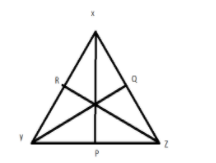QuestionAnswers

# The perimeter of a triangle is equal to K times the sum of its altitude, then K is:A)1B) any numberC) less than 1D)greater than 1

Hint: Perimeter is the sum of all the sides of the triangle. We will use formula of perimeter i.e. Perimeter=K × Sum of Altitudes.

Complete step-by-step solution:
Given: Perimeter of a triangle is equal to K times the sum of its altitude.
We know that, always, the perimeter of a triangle is greater than the sum of the altitudes.
Given, Perimeter=K × Sum of Altitudes
So, K is greater than 1.
Below is the proof that, always, the perimeter of a triangle is greater than the sum of the altitudes.
Let there be a △XYZ with its altitudes P, Q and R from the vertices X, Y and Z respectively.The altitudes form a right-angled triangle at their corresponding bases and in the right-angled triangle the hypotenuse is the longest side. Taking the right angles formed by the altitudes and the sides as the hypotenuse.
We observe that in each of the triangle, the side forms the longest side,
i.e.
In △XYP, XY is the longest side.
In △XZR, XZ is the longest side.
In △ZYP, YZ is the longest side.
So, adding all these three sides, we find that the perimeter of a triangle is greater than the sum of its three altitudes.
Hence, from the given multiple choices, option D is the correct answer.

Note: If we draw altitudes in a triangle the altitudes will be the longest segment in a triangle. And if we find the sum of altitude of a triangle.it should be less than the perimeter of the triangle.